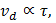# In the above question if potential difference is applied, the drift velocity at temperatureis a) Inversely proportional tob) Proportional toc) Zero d) Finite but independent of## Question ID - 150831 :- In the above question if potential difference is applied, the drift velocity at temperatureis a) Inversely proportional tob) Proportional toc) Zero d) Finite but independent of3537

 (a) When temperature is raised, the ions/atoms of the conductor start vibrating with increased amplitude of vibration and greater frequency. Due to which the electrons moving towards the positive end of conductor will suffermore rapid collisions and hence time of relaxationdecreaseds. Asthus drift velocity decrease. Therefore